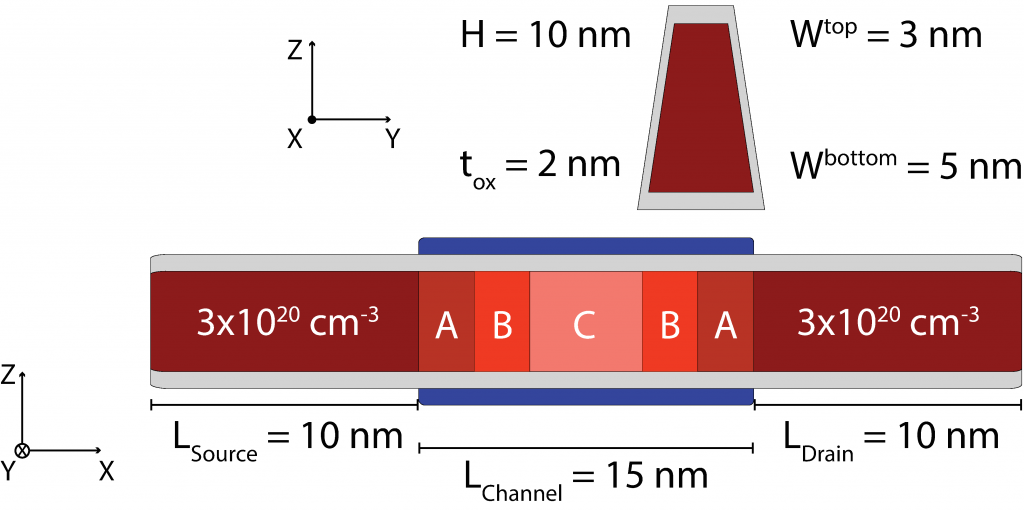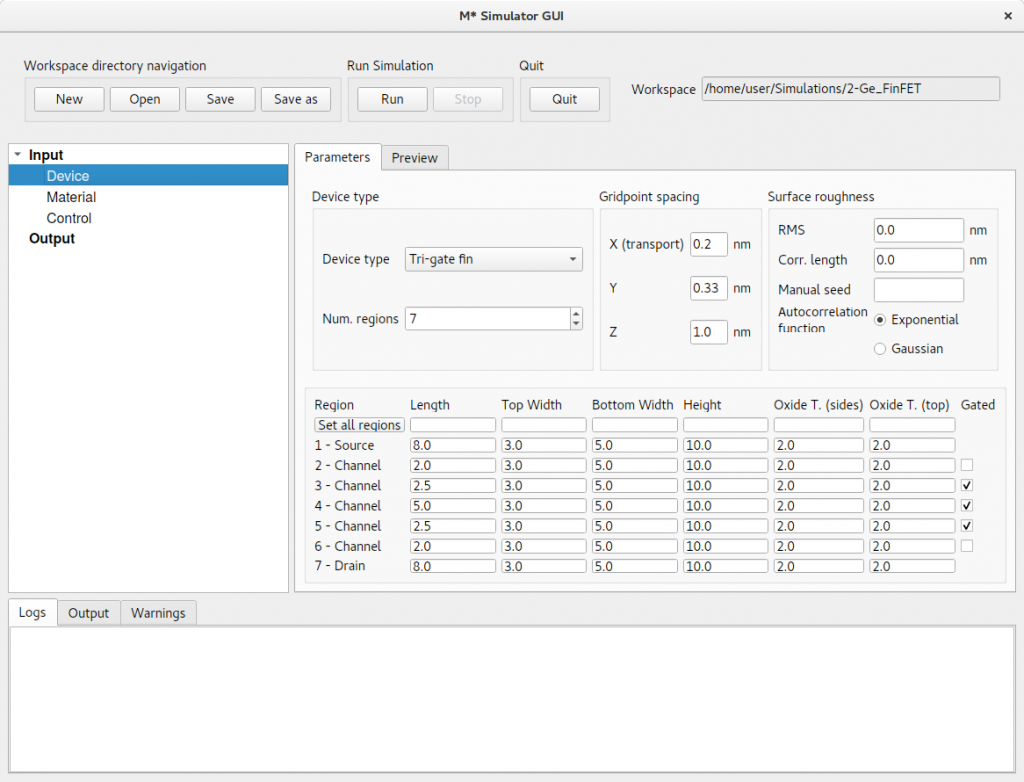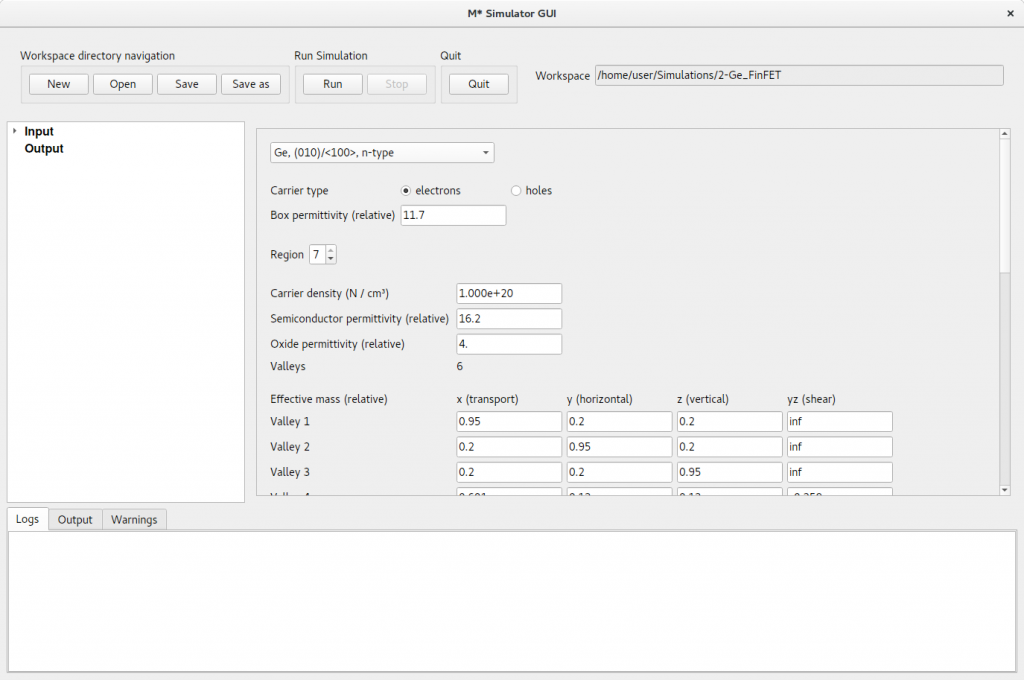# Tutorial: Germanium FinFETFigure 1:Ge FinFET: Schematic showing the device geometry. Red represents germanium, gray represents a low-κ dielectric, green a high-κ dielectric, yellow represents a silicon substrate, and blue the gate electrode. Regions in different shades of red represent variations in doping along the transport axis. The device is divided into several regions labelled A-D in order to describe dopant diffusion. In this tutorial we describe how to use M* to simulate a germanium fin field-effect-transistor (FinFET) in a tri-gate configuration using the UMS mode-space method. The device is comprised of a Ge 〈100〉 fin with constant trapezoidal cross-section along the transport direction with a height of 10 nm, a width of 5 nm at the base of the fin, and a width of 3 nm at the top of the fin. Figure 1 shows a schematic of the device geometry and doping profile. The device is divided into 7 regions in which we target varying carrier densities in order to model dopant diffusion into the channel: regions labelled A in the figure are doped to target a carrier concentration of 1 × 1020 cm-3, regions labelled B to 5 × 1019 cm-3, regions labelled C to 1 × 1018 cm-3, and region D to 1 × 1015 cm-3. In order to prepare the input files for simulating such a device, launch the M* GUI and populate the three input panels as follows:
1. Device select Tri-gate fin device type from the dropdown menu, set the number of regions to 7, and fill out the length of each region according to figure 1 and as shown in figure 2 below. Since this device’s cross-sectional geometry is homogeneous, you may specify the corresponding fields for all regions at once by typing in values in the first row and clicking Set all regions. Set regions 3, 4, and 5 as Gated. Finally, set the Gridpoint spacing parameters to 0.2 nm × 0.33 nm × 1.0 nm to reduce simulation time. Before continuing, check the Preview tab to visualise your input geometryFigure 2: In the Device panel, set the device type to Tri-gate fin with 7 regions and dimensions shown above. Make sure only regions 3 – 5 are Gated. Increase the gridpoint spacing to reduce simulation time.
2. Material set the material to Ge, (010)/〈100〉, n-type to set the semiconductor properties across the whole device. Cycle through regions 1 – 7 and set target carrier densities as described above for regions labelled A – D:
1. A: 1 × 1020 cm-3; εoxr = 4
2. B: 5 × 1019 cm-3; εoxr = 4
3. C: 1 × 1018 cm-3; εoxr = 20
4. D: 1 × 1015 cm-3; εoxr = 20
Additionally, set the oxide permittivity to εoxr = 4 to describe a low-κ dielectric covering non-gated regions, and a value εoxr =20 in regions 3 – 5 to simulate a high-κ oxide in gated regions. Set the buried oxide (BOX) permittivity to that of silicon (i.e. εboxr = 11.7) to simulate a device based on the aspect-ratio-trapping (ART) heteroepitaxy technique.Figure 3: In the Material panel, cycle through regions to set the material properties discussed in the text above.